﻿ Mathematics stock vector. Illustration of background - 12060654

# Mathematics.

## Illustration of mathematical equations and formulas on green background

Designers Also Selected These Stock IllustrationsAdorable future builder constructing a brick wallEducation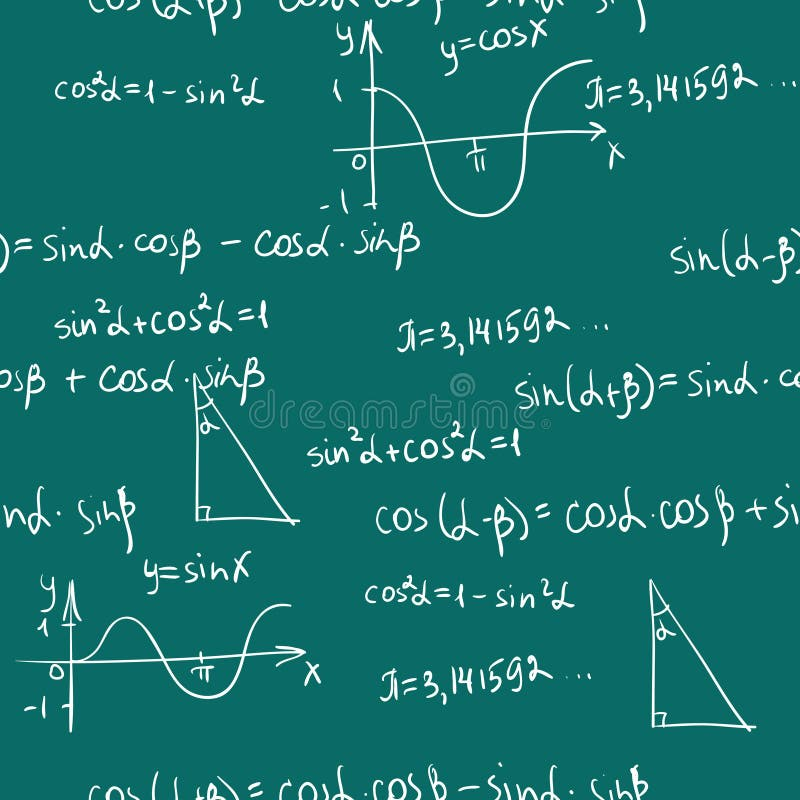Maths seamless pattern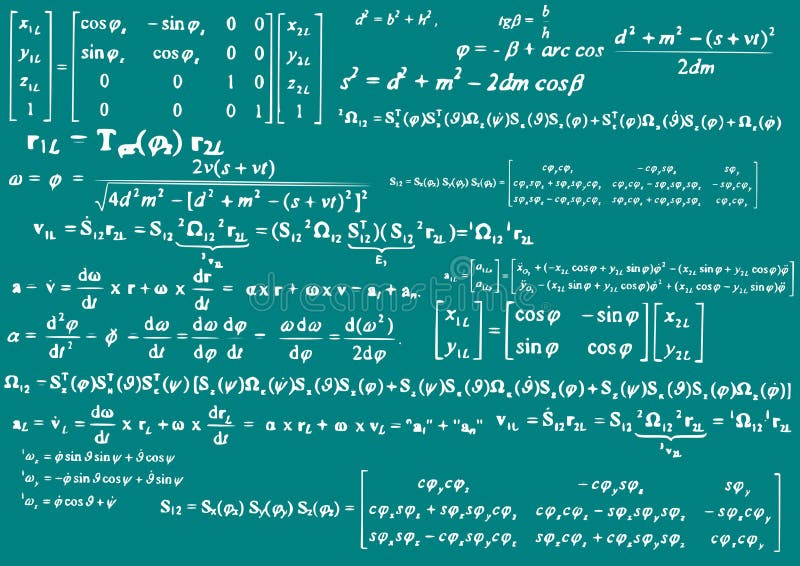Mathematics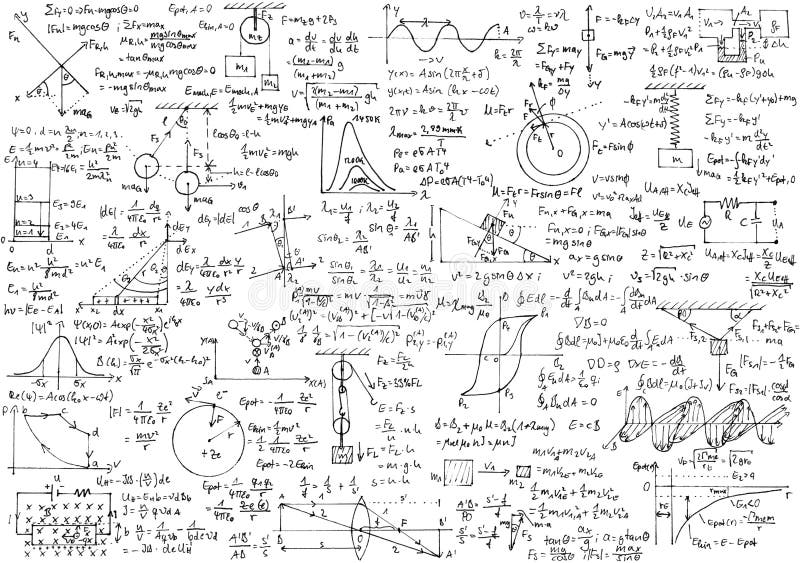Physics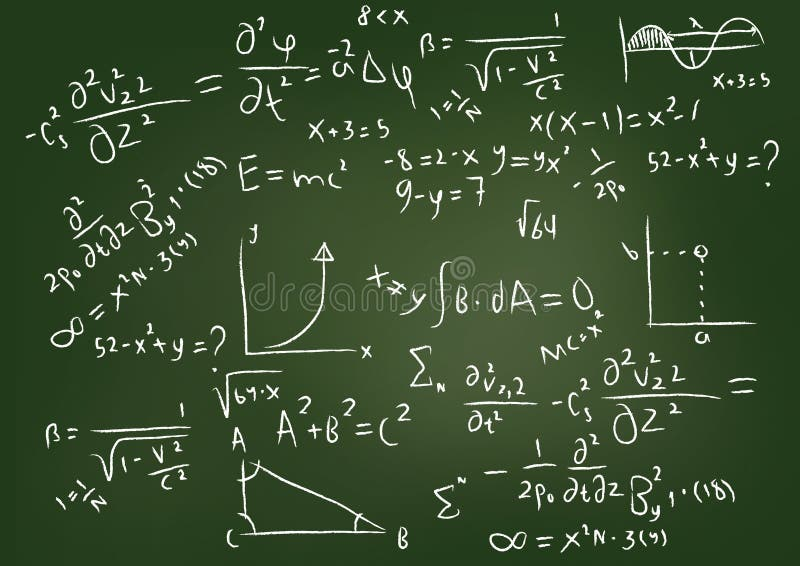EquationsConcept of heavy stress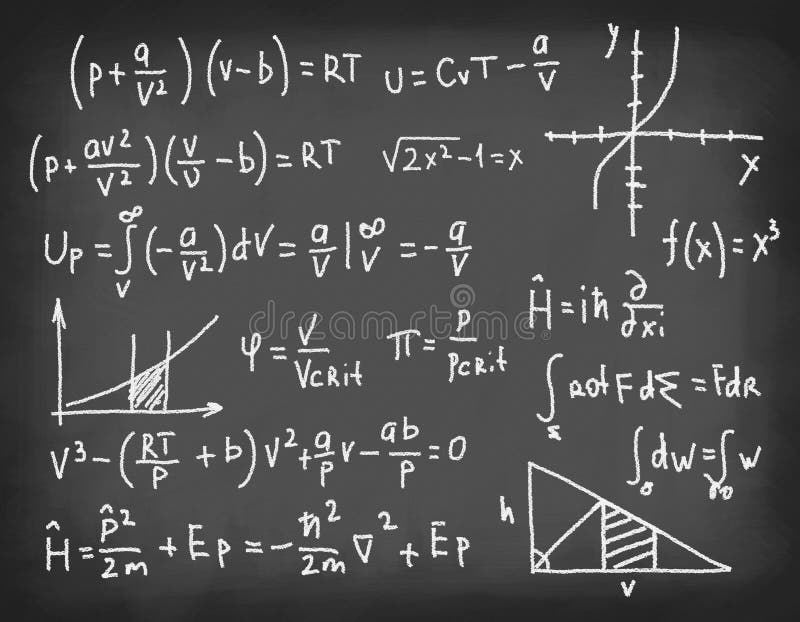Equations on blackboard.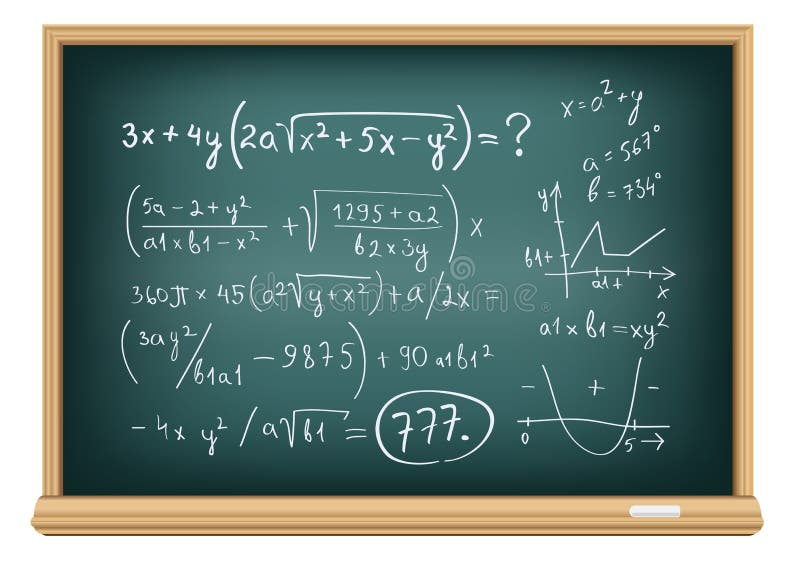Board difficult equations
More similar stock illustrations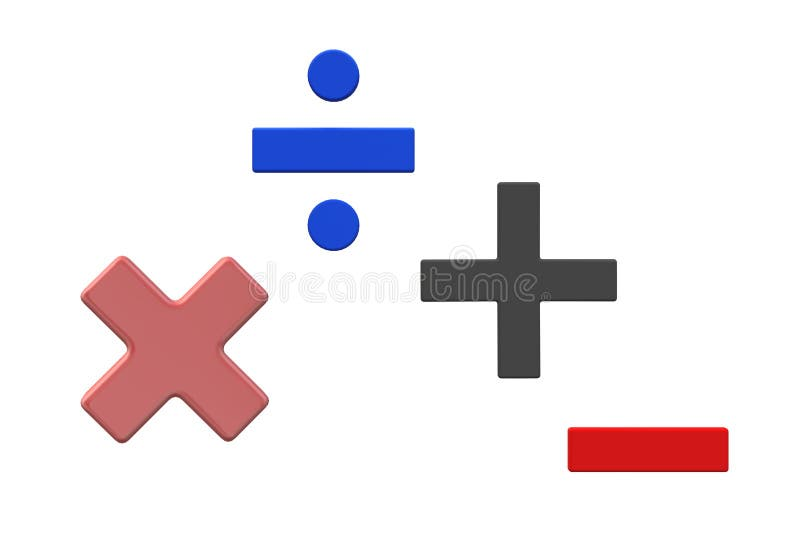Symbols of basic mathematics - multiplication, division, addition and subtraction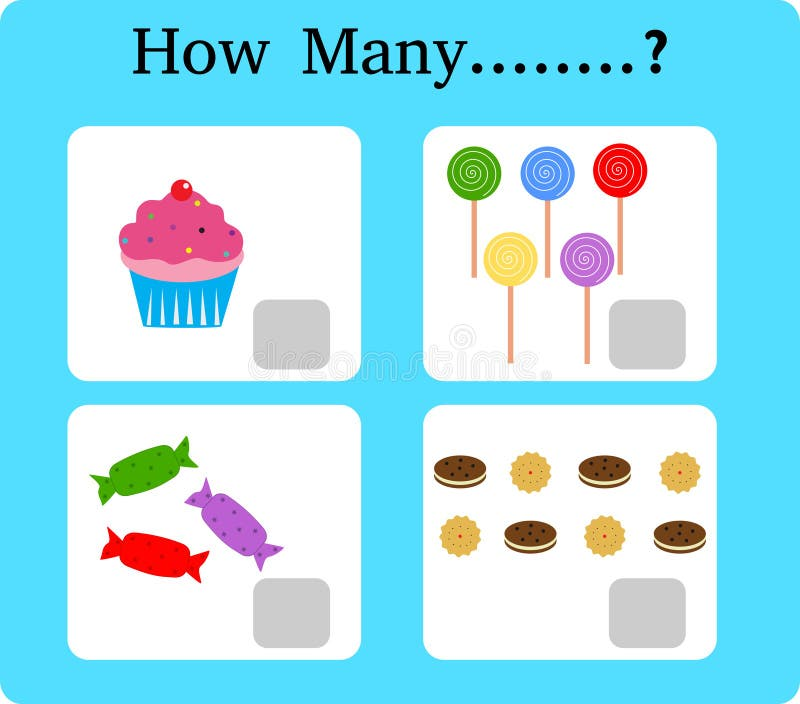Game for precounting, school children, game for kids, Learning mathematics, Educational a mathematical game, how many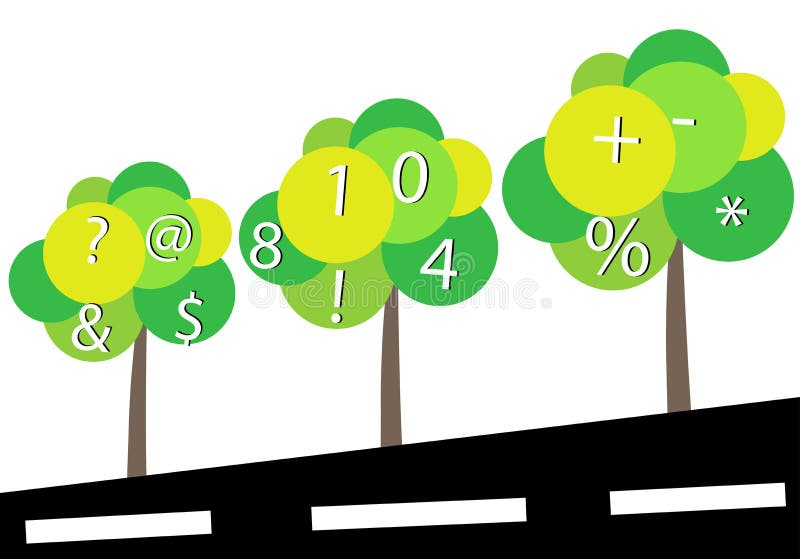Mathematics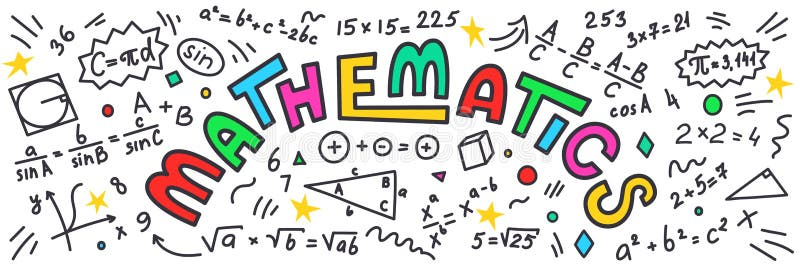Mathematics. Maths doodles with colorful lettering on white background.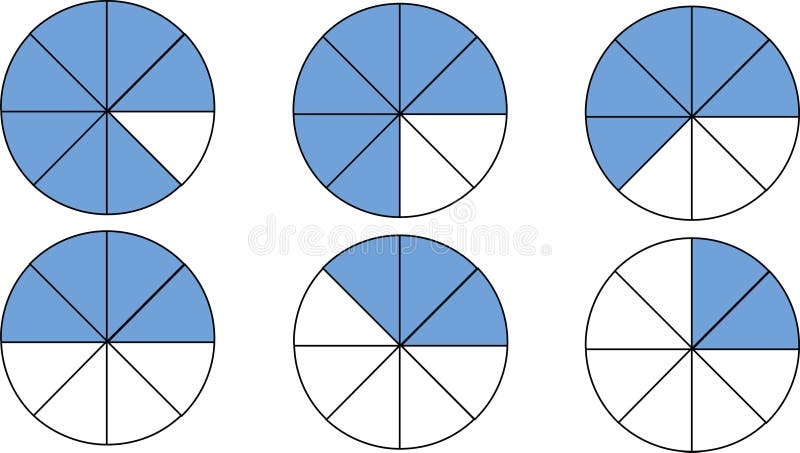Set of fractions. Mathematics. Fraction table to learn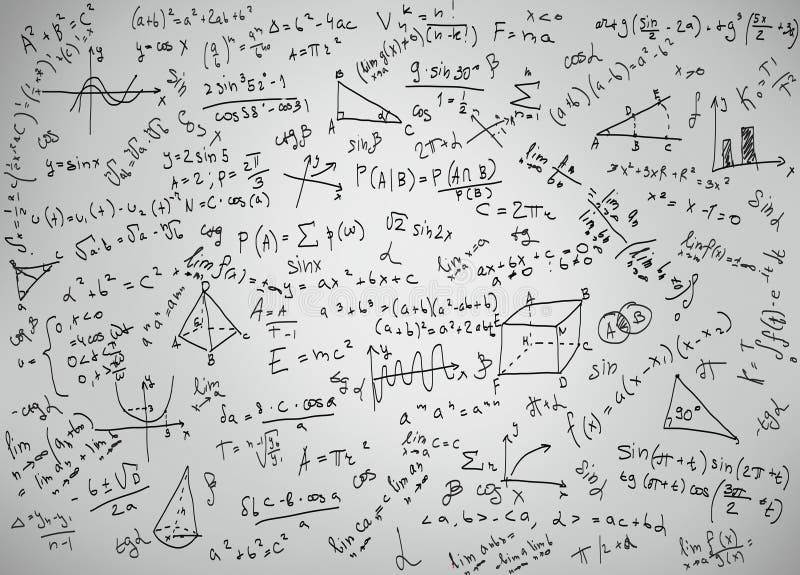Mathematics equations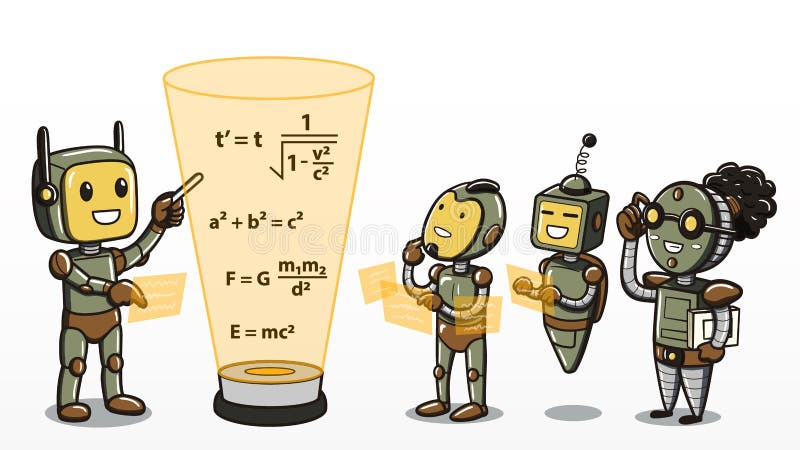Machine learning - Robots learning mathematics equations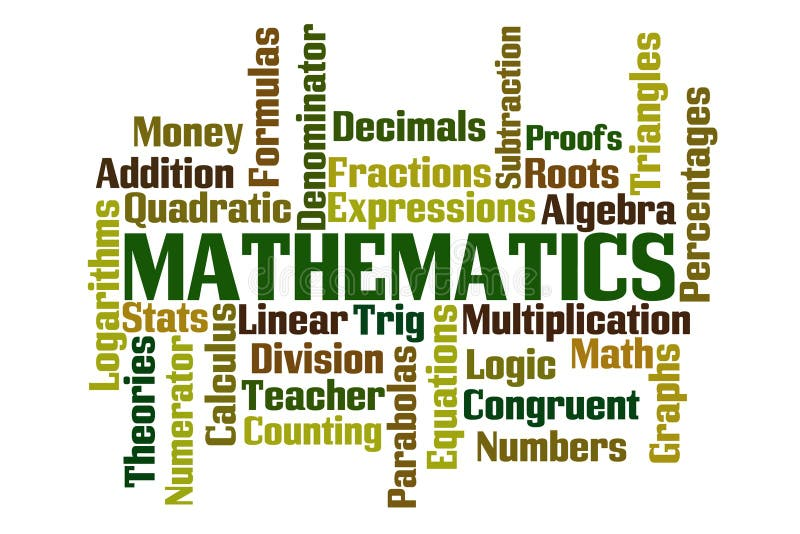Mathematics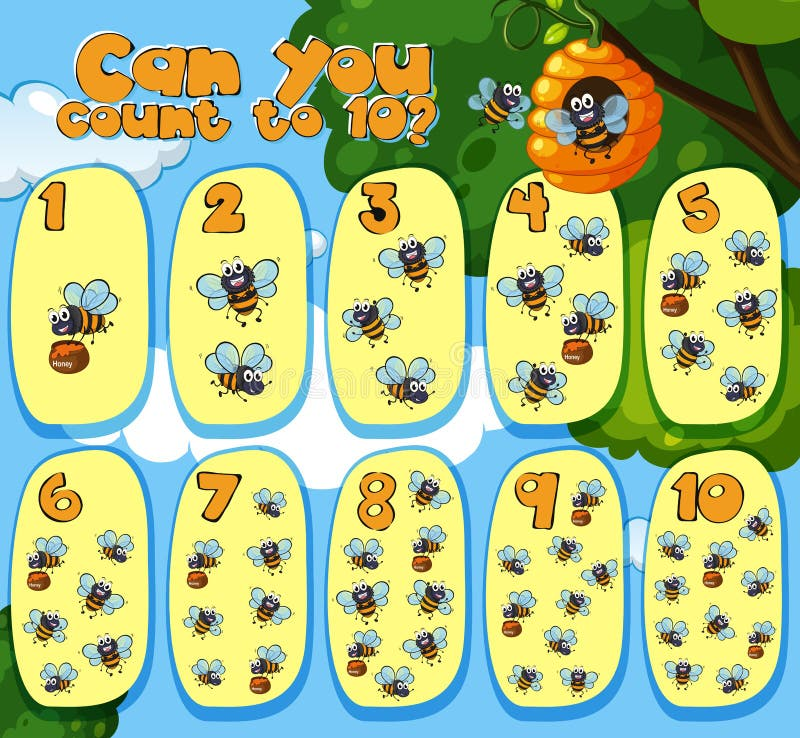Mathematics Counting Bees 1 to 10
More stock photos from Etiamos' portfolio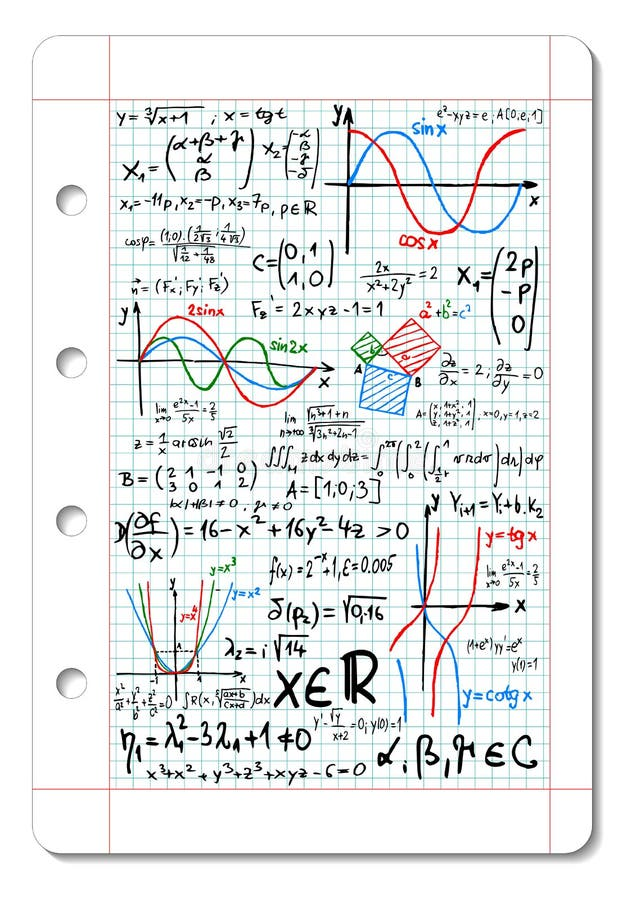Mathematics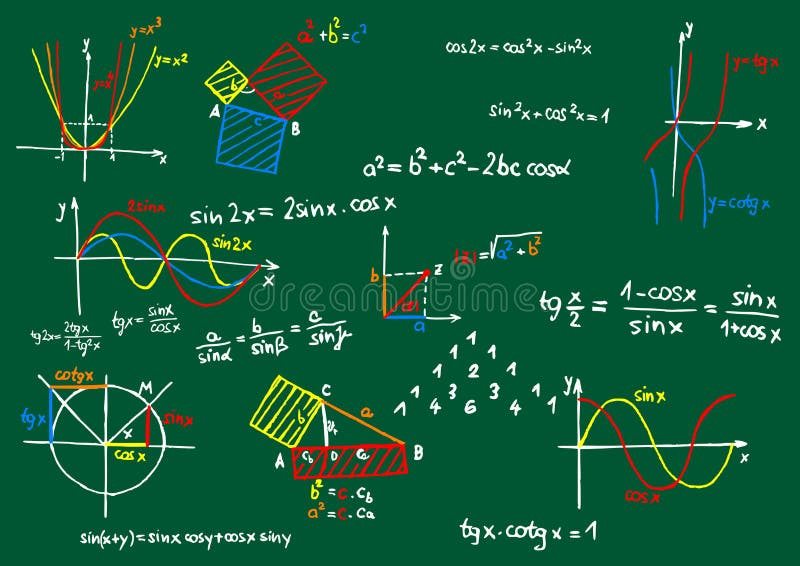Mathematics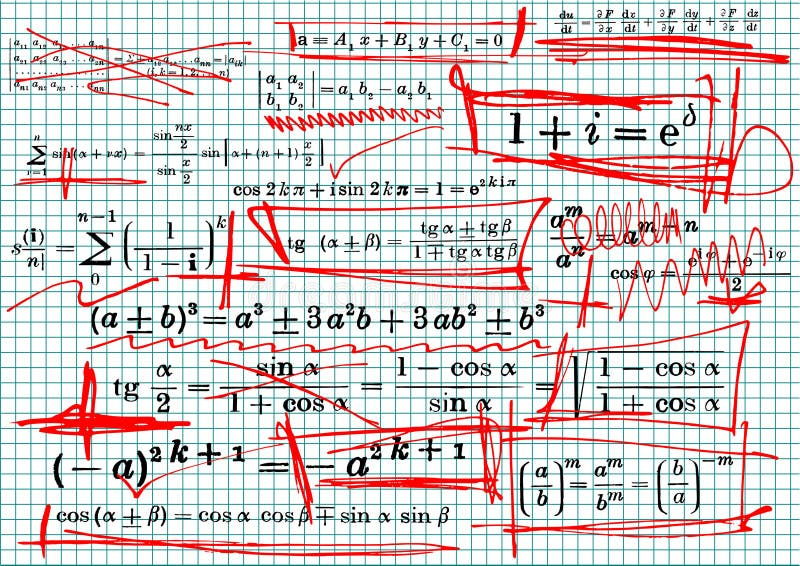Mathematics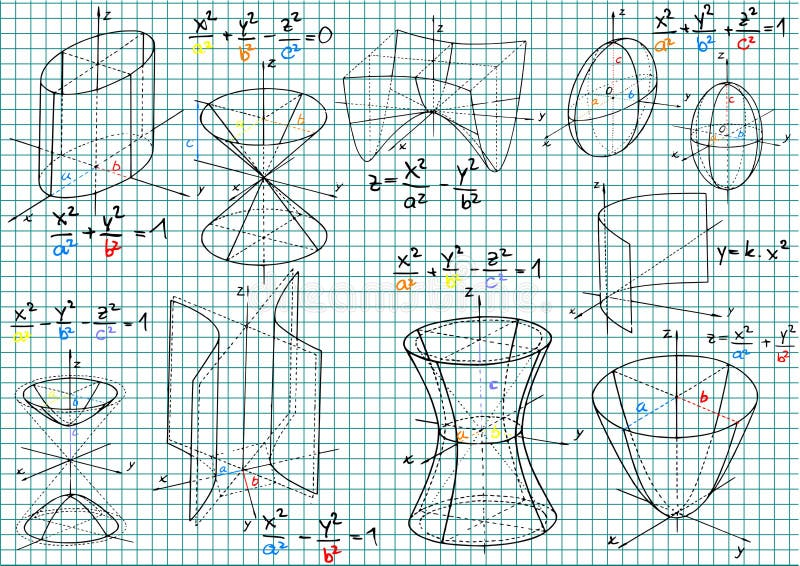Mathematics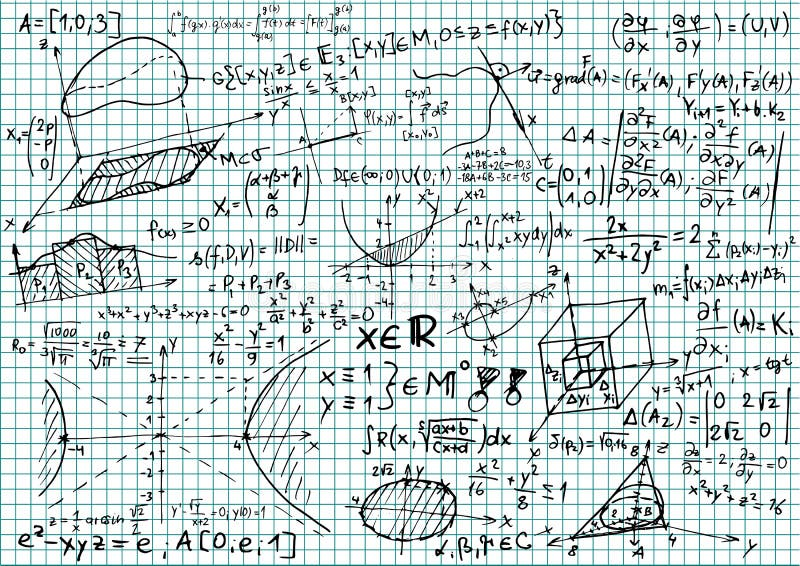Mathematics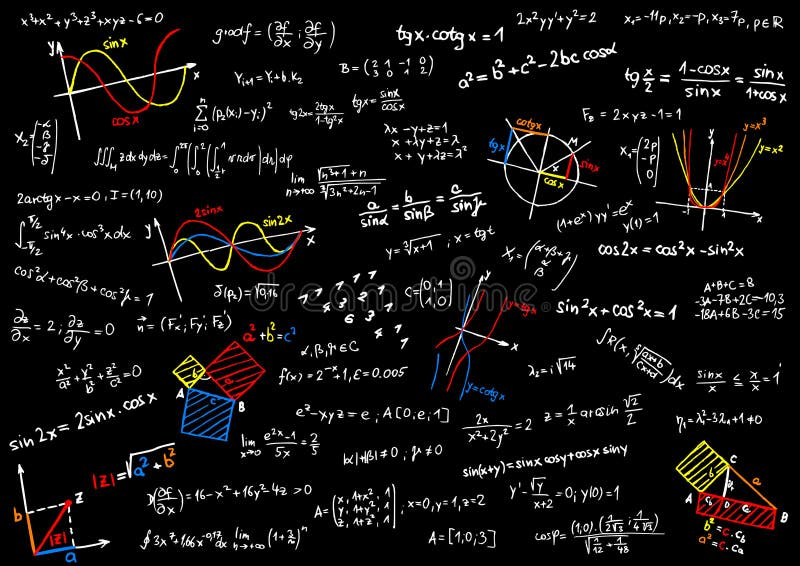Mathematics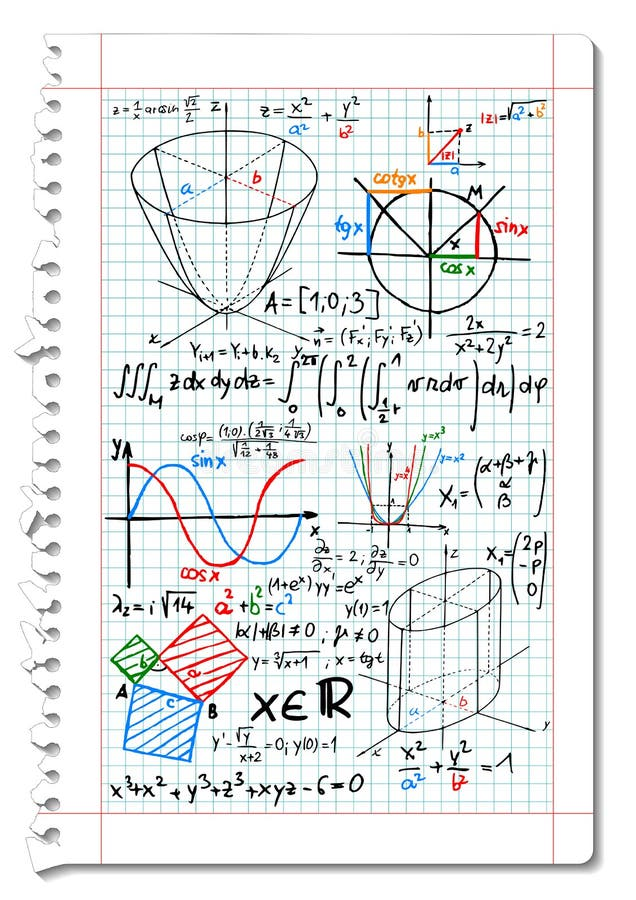Mathematics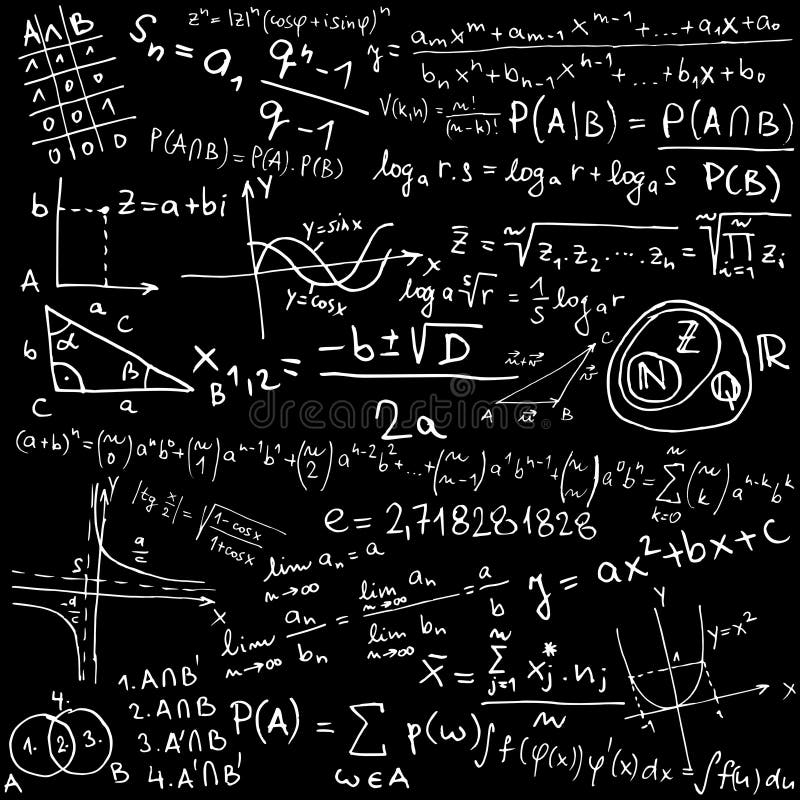Mathematics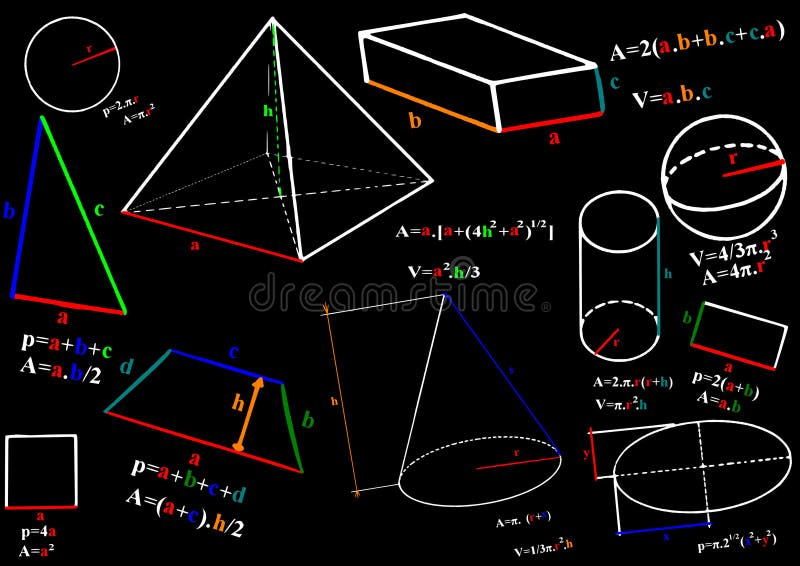Mathematics
Related categories
Extended licenses